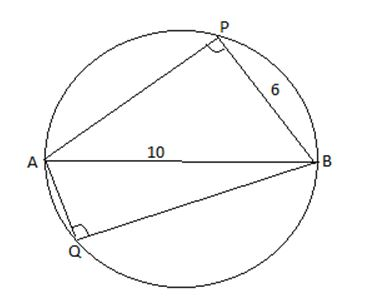# AB is a diameter of a circle of radius 5 cm | Quantitative Aptitude – Geometry – Mensuration

## CAT 2019 – Slot -1 - Question 5 - AB is a diameter of a circle of radius 5 cm...

Q. 5: AB is a diameter of a circle of radius 5 cm. Let P and Q be two points on the circle so that the length of PB is 6 cm, and the length of AP is twice that of AQ. Then the length, in cm, of QB is nearest to

1.  7.8
2.  8.5
3.  9.1
4.  9.3

See the figure,Given AB is diameter = 5*2 = 10 , BP =6 And AQ = AP/2

Angle P and Q will be right angled (angle in semi circle is always equals to 90 degree)

Thus in right angled triangle APB , AP = (AB^2 – BP^2)^1/2 = (10^2 – 6^2 )^1/2 = 8

So AQ = 8/2 = 4

Now in right angled triangle AQB, BQ = (AB^2 – AQ^2)^1/2 = (10^2 – 4^2 )^1/2 = (84)^1/2 = 9.1 approx.

### Past Year Question Paper & SolutionsCounselling Session
By IIM Mentor

#### Free Material Area